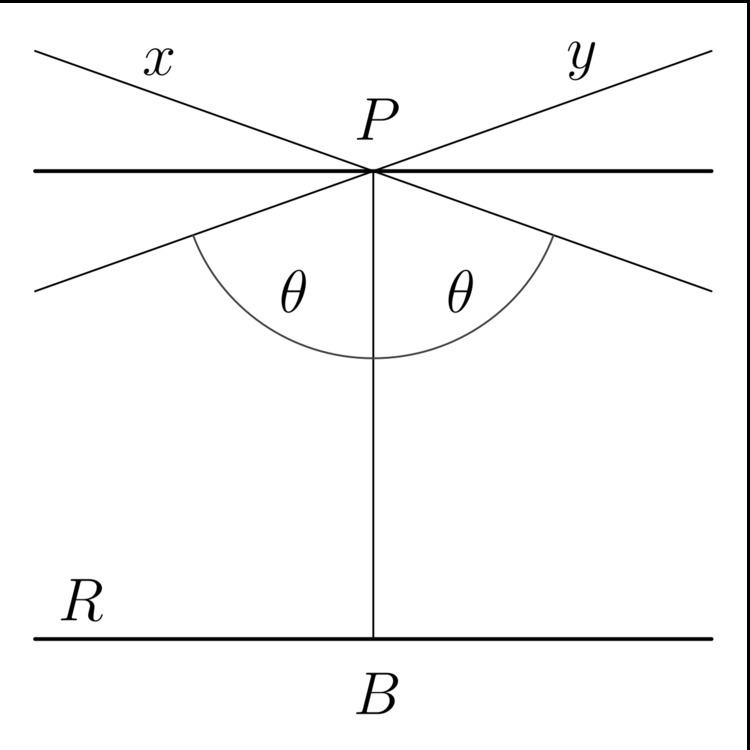# Limiting parallel

Updated on
Covid-19In neutral or absolute geometry, and in hyperbolic geometry, there may be many lines parallel to a given line l through a point P not on line R ; however, in the plane, two parallels may be closer to l than all others (one in each direction of R ).

## Contents

Thus it is useful to make a new definition concerning parallels in neutral geometry. If there are closest parallels to a given line they are known as the limiting parallel, asymptotic parallel or horoparallel (horo from Greek: ὅριον — border).

For rays, the relation of limiting parallel is an equivalence relation, which includes the equivalence relation of being coterminal.

Limiting parallels may form two, or three sides of a limit triangle.

## Definition

A ray A a is a limiting parallel to a ray B b if they are coterminal or if they lie on distinct lines not equal to the line A B , they do not meet, and every ray in the interior of the angle B A a meets the ray B b .

## Properties

Distinct lines carrying limiting parallel rays do not meet.

## Proof

Suppose that the lines carrying distinct parallel rays met. By definition the cannot meet on the side of A B which either a is on. Then they must meet on the side of A B opposite to a , call this point C . Thus C A B + C B A < 2  right angles a A B + b B A > 2  right angles . Contradiction.

Limiting parallel Wikipedia

Topics